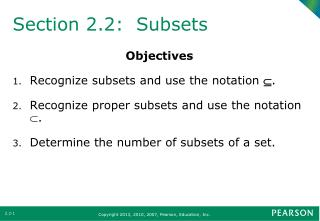Download PresentationSection 2.2: Subsets

# Section 2.2: Subsets - PowerPoint PPT PresentationDownload Presentation## Section 2.2: Subsets

- - - - - - - - - - - - - - - - - - - - - - - - - - - E N D - - - - - - - - - - - - - - - - - - - - - - - - - - -
##### Presentation Transcript

1. Section 2.2: Subsets Objectives • Recognize subsets and use the notation . • Recognize proper subsets and use the notation . • Determine the number of subsets of a set.

2. Subsets • Set A is a subset of set B, symbolized A ⊆ B, if and only if all elements of set A are also elements of set B. • The symbol A ⊆ B indicates that “set A is a subset of set B.” • The symbol A ⊈ B set A is not a subset of set B. • To show that set A is not a subset of set B, one must find at least one element of set A that is not an element of set B.

3. Determining Subsets Example: A = { 3, 5, 6, 8 } B = {1, 2, 3, 4, 5, 6, 7, 8, 9, 10} • Determine whether set A is a subset of set B. Solution: • Determine whether set B is a subset of set A. Solution:

4. Proper Subset • Set A is a proper subset of set B, symbolized A ⊂ B, if and only if all of the elements of set A are elements of set B and set A ≠ B. • (that is, set B must contain at least one element not is set A).

5. Determining Proper Subsets Example: A = { dog, cat } B = { dog, cat, bird, fish } • Determine whether set A is a proper subset of set B. Solution: • Determine whether set B is a proper subset of set A. Solution:

6. Determining Proper Subsets Example: Determine whether set A is a proper subset of set B. A = { dog, bird, fish, cat } B = { dog, cat, bird, fish } Solution:

7. Number of Distinct Subsets The number of distinct subsets of a finite set A is 2n, where n is the number of elements in set A. Example: • Determine the number of distinct subsets for the given set {t, a, p, e}. • List all the distinct subsets for the given set {t, a, p, e}.

8. Number of Distinct Proper Subsets The number of distinct proper subsets of a finite set A is 2n– 1, where n is the number of elements in set A. Example: Determine the number of distinct proper subsets for the given set{t, a, p, e}. Solution: